• 介绍online均值/方差统计算法在spark中的实现
1. 统计量

这个summarizer用于计算样本各维特征的均值，方差等常用统计量

class MultivariateOnlineSummarizer extends MultivariateStatisticalSummary with Serializable {

private var n = 0
//均值
private var currMean: Array[Double] = _
//用于方差统计
private var currM2n: Array[Double] = _
//平方和
private var currM2: Array[Double] = _
//L1范数
private var currL1: Array[Double] = _
//样本计数
private var totalCnt: Long = 0
//所有样本weight的和
private var totalWeightSum: Double = 0.0
//weight平方和，用于计算方差
private var weightSquareSum: Double = 0.0
//每维特征非0的权重和
private var weightSum: Array[Double] = _
//非0计数
private var nnz: Array[Long] = _
//最大值
private var currMax: Array[Double] = _
//最小值
private var currMin: Array[Double] = _

2. online统计

这里的统计项，除了均值和方差，其他的直接计算即可， Wikipedia给出了带权online统计算法，这里的算法支持分布式统计，各部分样本先合并，然后各个统计器再合并。样本统计

EN=EN−1+wN∑Ni=0wi(x−EN−1)⋯⋯(1)SN=SN−1+wN∗(x−EN−1)(x−EN)⋯⋯(2)<!--//--><![CDATA[//><!--
E_{N}=E_{N-1}+\frac{w_N}{\sum_{i=0}^N wi} (x-E_{N-1})   \cdots\cdots (1) \\
S_N=S_{N-1}+w_{N}*(x-E_{N-1})(x-E_N)  \cdots\cdots (2)
//--><!]]>EN<!--//--><![CDATA[//><!--
E_N
//--><!]]>，SN<!--//--><![CDATA[//><!--
S_N
//--><!]]>，wN<!--//--><![CDATA[//><!--
w_N
//--><!]]>表示第N个样本的均值，方差，权重，x是样本值，具体实现在add函数中

private[spark] def add(instance: Vector, weight: Double): this.type = {
require(weight >= 0.0, s"sample weight, ${weight} has to be >= 0.0") if (weight == 0.0) return this if (n == 0) { require(instance.size > 0, s"Vector should have dimension larger than zero.") //输入特征向量维度 n = instance.size //初次分配空间 currMean = Array.ofDim[Double](n) currM2n = Array.ofDim[Double](n) currM2 = Array.ofDim[Double](n) currL1 = Array.ofDim[Double](n) weightSum = Array.ofDim[Double](n) nnz = Array.ofDim[Long](n) currMax = Array.fill[Double](n)(Double.MinValue) currMin = Array.fill[Double](n)(Double.MaxValue) } require(n == instance.size, s"Dimensions mismatch when adding new sample." + s" Expecting$n but got ${instance.size}.") val localCurrMean = currMean val localCurrM2n = currM2n val localCurrM2 = currM2 val localCurrL1 = currL1 val localWeightSum = weightSum val localNumNonzeros = nnz val localCurrMax = currMax val localCurrMin = currMin //迭代每一维特征 instance.foreachActive { (index, value) => //仅统计非0部分，特别在特征稀疏的时候，减少计算量 if (value != 0.0) { //max，min if (localCurrMax(index) < value) { localCurrMax(index) = value } if (localCurrMin(index) > value) { localCurrMin(index) = value } val prevMean = localCurrMean(index) val diff = value - prevMean //式(1) localCurrMean(index) = prevMean + weight * diff / (localWeightSum(index) + weight) //式(2) localCurrM2n(index) += weight * (value - localCurrMean(index)) * diff //平方和 localCurrM2(index) += weight * value * value //L1范数 localCurrL1(index) += weight * math.abs(value) localWeightSum(index) += weight localNumNonzeros(index) += 1 } } totalWeightSum += weight weightSquareSum += weight * weight totalCnt += 1 this } 两个统计器之间的合并，如果是带权重的，n就是权重和，A、B是两个待合并的统计器，x是合并后的 nx=nA+nB⋯⋯(3)δ=EB−EA⋯⋯(4)Ex=EA+δ∗nBnx⋯⋯(5)Sx=SA+SB+δ2∗nA∗nBnx⋯⋯(6)<!--//--><![CDATA[//><!-- n_x = n_A+n_B \cdots\cdots (3) \\ \delta = E_B-E_A \cdots\cdots (4) \\ E_x = E_A + \delta * \frac{n_B}{n_x} \cdots\cdots (5) \\ S_x=S_A+S_B+\delta^2*\frac{n_A*n_B}{n_x} \cdots\cdots (6) //--><!]]> 因此spark的实现 @Since("1.1.0") def merge(other: MultivariateOnlineSummarizer): this.type = { if (this.totalWeightSum != 0.0 && other.totalWeightSum != 0.0) { require(n == other.n, s"Dimensions mismatch when merging with another summarizer. " + s"Expecting$n but got \${other.n}.")
totalCnt += other.totalCnt
totalWeightSum += other.totalWeightSum
weightSquareSum += other.weightSquareSum
var i = 0
while (i < n) {
val thisNnz = weightSum(i)
val otherNnz = other.weightSum(i)
//式(3)
val totalNnz = thisNnz + otherNnz
val totalCnnz = nnz(i) + other.nnz(i)
if (totalNnz != 0.0) {
//式(4)
val deltaMean = other.currMean(i) - currMean(i)
// merge mean together， 式(5)
currMean(i) += deltaMean * otherNnz / totalNnz
// merge m2n together，式(6)
currM2n(i) += other.currM2n(i) + deltaMean * deltaMean * thisNnz * otherNnz / totalNnz
// merge m2 together
currM2(i) += other.currM2(i)
// merge l1 together
currL1(i) += other.currL1(i)
// merge max and min
currMax(i) = math.max(currMax(i), other.currMax(i))
currMin(i) = math.min(currMin(i), other.currMin(i))
}
weightSum(i) = totalNnz
nnz(i) = totalCnnz
i += 1
}
} else if (totalWeightSum == 0.0 && other.totalWeightSum != 0.0) {
this.n = other.n
this.currMean = other.currMean.clone()
this.currM2n = other.currM2n.clone()
this.currM2 = other.currM2.clone()
this.currL1 = other.currL1.clone()
this.totalCnt = other.totalCnt
this.totalWeightSum = other.totalWeightSum
this.weightSquareSum = other.weightSquareSum
this.weightSum = other.weightSum.clone()
this.nnz = other.nnz.clone()
this.currMax = other.currMax.clone()
this.currMin = other.currMin.clone()
}
this
}

3. 统计量计算

从代码中可以看到，均值和方差的计算都只计算了非0部分的样本，在返回时，需要计算入为0的部分。这部分样本实际相当于方差和均值都是0的统计器，与非0部分merge。

3.1. 均值

还是套用上面merge的公式，NZ是非0部分，Z代表样本值为0的部分 δ=ENZ−EZ=ENZ⋯⋯(7)Eall=EZ+δ∗nNZnx=ENZ∗∑NZwi∑allwi⋯⋯(8)<!--//--><![CDATA[//><!--
\begin{aligned}
\delta = E_{NZ}-E_Z=E_{NZ}\cdots\cdots (7) \\
E_{all} = E_Z+\delta*\frac{n_{NZ}}{n_x}=E_{NZ}*\frac{\sum_{NZ}w_i}{\sum_{all}w_i}\cdots\cdots (8)
\end{aligned}
//--><!]]>
对应代码实现

override def mean: Vector = {
require(totalWeightSum > 0, s"Nothing has been added to this summarizer.")

val realMean = Array.ofDim[Double](n)
var i = 0
while (i < n) {
//式(8)
realMean(i) = currMean(i) * (weightSum(i) / totalWeightSum)
i += 1
}
Vectors.dense(realMean)
}

3.2. 方差

同理，对于方差来说

Sx=SZ+SNZ+δ2∗nA∗nBnx=SNZ+E2NZ∗∑Zwi∗∑NZwi∑allwi⋯⋯(9)<!--//--><![CDATA[//><!--
\begin{aligned}
S_x  &=S_{Z}+S_{NZ}+\delta^2*\frac{n_A*n_B}{n_x}  \\
&=S_{NZ}+E_{NZ}^2*\frac{\sum_{Z}w_i*\sum_{NZ}w_i}{\sum_{all}wi}\cdots\cdots (9)
\end{aligned}
//--><!]]>
又由Wikipedia可知，对于Reliability Weights来说，其方差

d=∑wi−∑w2i/∑wi⋯⋯(10)Sr=Sd⋯⋯(11)<!--//--><![CDATA[//><!--
d=\sum w_i-\sum w_i^2/\sum w_i\cdots\cdots (10)\\
S_r=\frac{S}{d}\cdots\cdots (11)
//--><!]]>
因此其代码实现

override def variance: Vector = {
require(totalWeightSum > 0, s"Nothing has been added to this summarizer.")

val realVariance = Array.ofDim[Double](n)
//式(10)，d
val denominator = totalWeightSum - (weightSquareSum / totalWeightSum)

// Sample variance is computed, if the denominator is less than 0, the variance is just 0.
if (denominator > 0.0) {
val deltaMean = currMean
var i = 0
val len = currM2n.length
while (i < len) {
//分子式(9)，整体式(11)
realVariance(i) = (currM2n(i) + deltaMean(i) * deltaMean(i) * weightSum(i) *
(totalWeightSum - weightSum(i)) / totalWeightSum) / denominator
i += 1
}
}
Vectors.dense(realVariance)
}

currM2n为online计算得到的S，deltaMean为均值，第二项就是上式中的第二项，为0的权重和使用所有权重减去非0的权重和得到的，再除以denominator。

3.3. L2范数

∥x∥2=∑ix2i−−−−−√<!--//--><![CDATA[//><!--
\parallel x\parallel _2=\sqrt{\sum_i x_i^2}
//--><!]]>
我们统计时计算了平方和，因此只需要取平方根，对应源码

override def normL2: Vector = {
require(totalWeightSum > 0, s"Nothing has been added to this summarizer.")

val realMagnitude = Array.ofDim[Double](n)

var i = 0
val len = currM2.length
while (i < len) {
realMagnitude(i) = math.sqrt(currM2(i))
i += 1
}
Vectors.dense(realMagnitude)
}

3.3. L1范数

∥x∥1=∑i∣xi∣<!--//--><![CDATA[//><!--
\parallel x\parallel _1=\sum_i \mid x_i\mid
//--><!]]>
源码

override def normL1: Vector = {
require(totalWeightSum > 0, s"Nothing has been added to this summarizer.")

Vectors.dense(currL1)
}

3.3. 其他统计项

同时统计了样本数量count，各维特征非0个数（numNonzeros，Vector），max/min(Vector)，直接返回对应值即可
展开全文spark
• # -*- coding: utf-8 -*- import os import cv2 import numpy as np def compute_mean(path): file_names = os.listdir(path) file_names.sort() per_image_Rmean = [] per_image_Gmean = [] ...
# -*- coding: utf-8 -*-

import os
import cv2
import numpy as np

def compute_mean(path):
file_names = os.listdir(path)
file_names.sort()

per_image_Rmean = []
per_image_Gmean = []
per_image_Bmean = []

image_R_std = []
image_G_std = []
image_B_std = []
// 我这里是两个子文件夹
for file in file_names:
file_path = os.path.join(path, file)
img_list = os.listdir(file_path)
img_list.sort()
for img_path in img_list:
img_ = os.path.join(file_path, img_path)
print(img_)

img = cv2.imread(img_)
per_image_Bmean.append(np.mean(img[:, :, 0] / 255.0))
per_image_Gmean.append(np.mean(img[:, :, 1] / 255.0))
per_image_Rmean.append(np.mean(img[:, :, 2] / 255.0))

image_B_std.append(np.std(img[:, :, 0] / 255.0))
image_G_std.append(np.std(img[:, :, 1] / 255.0))
image_R_std.append(np.std(img[:, :, 2] / 255.0))

R_mean = np.mean(per_image_Rmean)
G_mean = np.mean(per_image_Gmean)
B_mean = np.mean(per_image_Bmean)

R_std = np.std(image_R_std)
G_std = np.std(image_G_std)
B_std = np.std(image_B_std)
return R_mean, G_mean, B_mean, R_std, G_std, B_std

def main():
path = '/home/Disc_Z/youku/train/LR'
R_mean, G_mean, B_mean, R_std, G_std, B_std = compute_mean(path)
print('R_mean', R_mean)
print('G_mean', G_mean)
print('B_mean', B_mean)

print('R_std', R_std)
print('G_std', G_std)
print('B_std', B_std)

if __name__ == '__main__':
main()



展开全文• 做了几道概率统计题，整理分布~ 第一题 美国房地产协会报道了美国房屋价格的中位数和 5年期间房屋价格中位数的增长率（《华尔街日报》．2006 年 1 月 16 日）。利用下面房屋价格（单位：1000 美元）的样本数据...
做了几道概率统计题，整理分布~

第一题

美国房地产协会报道了美国房屋价格的中位数和 5年期间房屋价格中位数的增长率（《华尔街日报》．2006 年 1 月 16 日）。利用下面房屋价格（单位：1000 美元）的样本数据回答下列问题

995. 9  48. 8  175. 0  263. 5  298. 0  218. 9  209. 0

628.3  111.0  212.9  92.6  2325.0  958.0  212.5

a. 房屋价格样本中位数是多少？

215.9

b. 在 2001 年 1 月，美国房地产协会报道了美国房屋价格的中位数为 139 300 美元。5年期间房屋价格中位数增长率是多少？

54.99%

c. 样本数据的第一四分位数和第三四分位数是多少？

Q1=183.5 ； Q3=545.725

老师答案是 c.    175.0, 628.3

d. 对房屋价格应用五数概括法。

min     48.80000

25%     183.50000

50%     215.90000

75%     545.72500

max      2325.00000

e. 数据中有异常值吗？

异常值是指为大于Q3+1.5IQR或小于Q1−1.5IQR的值,区间为-359.84 ~ 1089.06。

共有1个异常值： 2325.0，

f. 房屋价格的样本均值是多少？为什么美国房地产协会在报道中更喜欢使用房屋价格的中位数？

样本均值 482.10

当存在异常值的时候，中位数可以提供比平均数更好的中心位置度量。

平均数因受异常值的影响，经常会被夸大或大幅降低。

第二题

位于亚特兰大市哈茨菲尔德－杰克逊机场的书亭出售报刊（平装书、报纸和杂志）和零食（花生、椒盐卷饼和糖果等）。销售点终端 (POS) 中收集了消费者大量的购买信息。下表中给出了最近 600名顾客购买零食和报刊的数量。

阅读材料

0

1

2

零食

0

0

60

18

1

240

90

30

2

120

30

12

1. 随机选取一名消费者，令 x= 零食购买量，y=报刊购买量。根据表中数据，求 x 和 y 的经验离散型二元概率分布。求顾客购买 1 种报刊和 2种零食的概率。求顾客只购买 1 种零食的概率。概率 f(x=O, y=O) =0, 为什么？

x,y的二元经验离散概率分布

x=零食购买量

y=报刊购买量

合计

0

1

2

0

0.00

0.10

0.03

0.13

1

0.40

0.15

0.05

0.60

2

0.20

0.05

0.02

0.27

合计

0.60

0.30

0.10

1.00

顾客购买 1 种报刊和 2种零食的概率:

f(x=2,y=1) = 0.05

顾客只购买 1 种零食的概率:

这里有歧义，如果是指只够买1种零食，不购买报刊，概率为0.40

如果是指只够买1种零食，不考虑报刊购买量，概率为0.60

概率 f(x=0, y=0) =0, 为什么？

因为光顾书亭的顾客，没有人既不消费零食又不消费报刊，是没有发生的事件。

2. 给出零食购买量的边际概率分布，并求其数学期望和标准差。

零食购买量的边际概率分布：

x=零食购买量

0

1

2

P

0.13

0.6

0.27

数学期望E(x)=0*0.13+1*0.6+2*0.27=1.14

标准差 σx  = 0.6168

3. 求报刊购买量的数学期望和标准差。

数学期望E(y)=0.5     标准差 σy  = 0.6708

4. 令 t= 零食和报刊购买量之和，给出 t 的概率分布，并求其数学期望和标准差。

t 的概率分布：

t=x+y

1

2

3

4

p

0.50

0.38

0.10

0.02

数学期望E(t)=1.64     标准差 σt  = 0.7419

5. 计算 x 和 y 的协方差和相关系数。顾客购买零食数量和报刊数量之间的关系如何？

x 和 y协方差：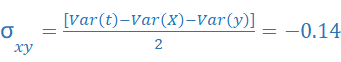相关系数: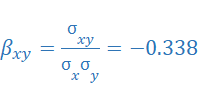相关系统为-0.338,表明零食数量和报刊数量之间是负相关的关系。

第三题

假设某大学入学考试的考试成绩服从正态分布．其均值为 450, 标准差为 100 。

1. 考试分数在 400 -500 的人数占多大百分比？

考试成绩x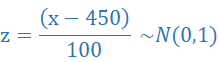P(400≤x≤500)=P（-0.5≤z≤0.5）=2*0.6915-1=0.383

所以，有38.3%的考试分数在400-500间。

2. 假定某人得分 630, 比此人考试分数高的考生的百分比有多大？比此人考试分数低的考生的百分比有多大？

P(x≥630)=1-P(x≤630)=1-P(z≤1.8)=1-0.9641=0.0359

比此人考试分数630分高的考生的百分比3.59%

比此人考试分数630分低的考生的百分比96.41%

3. 如果某大学不招收分数在 480 分以下的学生，参加考试的学生中被该大学接受的百分比是多少？

P(x≥480)=1-P(x≤480)=1-P(z≤0.3)=1-0.6179=0.3821

参加考试的学生中被该大学接受的百分比是38.21%

第四题

美国 50 岁及以上年龄的人口达 9200 万，他们掌握了整个可支配收入的 50% (AARP B1dletin, 2008年 3 月）。据美国退休人员协会 (AARP) 估计，在这一年龄段食堂和外卖的年人均消费为 1837美元。假定样本由 80 名民众组成，并且样本标准差为 550 美元。

1. 当置信度为 95 % 时，边际误差为多大？

用x表示50 岁及以上年龄食堂和外卖的年人均消费，在总体标准差未知情况下，用样本标准差s来估计σ，边际误差和总体均值的区间估计服从t分布。

边际误差为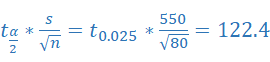2. 求用于食堂和外卖上的消费额的总体均值的95 % 置信区间。

95 % 置信区间: 1837 ± 122.4，即1714.6~1959.4

3. 估计美国 50 岁及以上年龄的人用于食堂和外卖上的消费是多少？

美国 50 岁及以上年龄的人口达 9200 万，在这一年龄段食堂和外卖的年人均消费为 1837美元，该群体总消费为9200 *1837万美元=1690.04亿美元

4. 如果食堂和外卖上的消费额是右偏的，那么你预计消费额的 中位数是大于还是小于1837美元？

右偏是指众数偏左，长尾偏右的图形，中位数肯定是小于1837。

第五题

美国个人投资者协会的在线折扣经纪商调查，是根据协会会员与折扣经纪商的经验进行的一项调查工作 。作为调查的一部分，要求 AAII 的会员对经纪商的执行速度能力作出评估，以及对电子交易提供一个整体满意度等级 。 可能的回答（分数）分别为无意见(O)， 不满意 (1). 比较满意 (2), 满意 (3). 非常满意 (4) 。 根据每个受访者提供的分数，计算出加权平均数，得到每位经纪商的总分数 。 部分调查结果如下表所示 (AAII website, 2012 年 2 月 7 日） 。

经纪公司

速度

满意度

A

3.4

3.5

B

3.3

3.4

C

3.4

3.9

D

3.6

3.7

E

3.2

2.9

F

3.8

2.8

G

3.8

3.6

H

2.6

2.6

I

2.7

2.3

J

4.0

4.0

K

2.5

2.5

a 以执行速度为自变量，绘制出这些数据的散点图 。

import pandas as pd

import matplotlib.pyplot as plt

df = pd.read_csv("survey.csv")

plt.scatter(df["Speed"],df["Satisfaction"])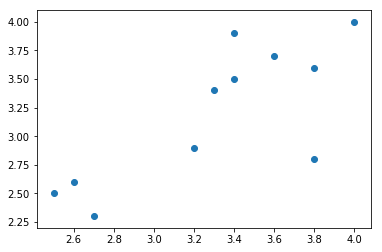b. 根据在 (a) 中作出的散点图，这两个变量之间显示出什么关系？

两个变量之间总体呈现正相关线性关系，当执行速度越快时，电子交易整体满意度越高。

c. 利用最小二乘法，建立估计的回归方程 。

import statsmodels.api as sm

from statsmodels.formula.api import ols

survey_model = ols("Satisfaction ~Speed",data=df).fit()

print(survey_model.summary())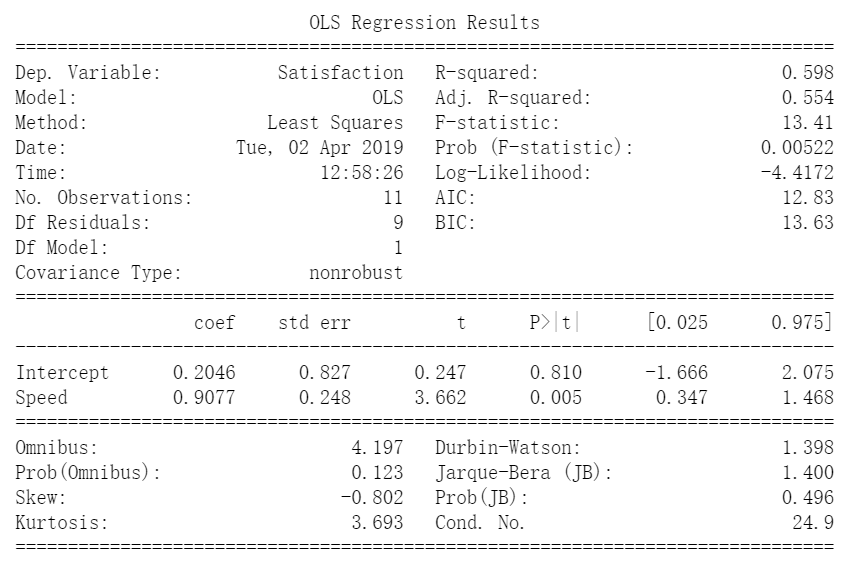估计的回归方程为 Satisfaction = 0.2046 + 0.9077 * Speed

这题利用excel做出散点图，添加趋势线，趋势线选项中勾选显示公式，R2值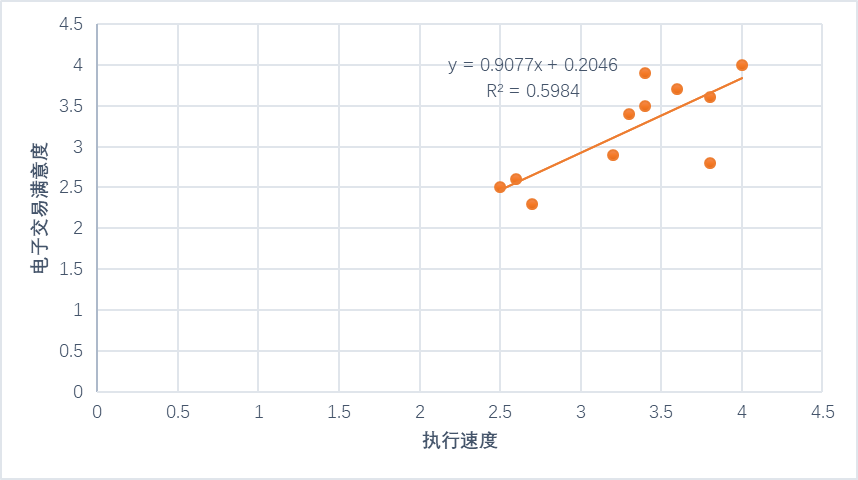d. 对估计的回归方程的斜率作出解释 。

回归方程的斜率为0.9077，说明执行速度同交易满意度是呈正相关的。每增加一个单位的执行速度分数，交易满意度等级增加0.9077个单位。

f. 假定 M 开发了新软件提高了它们执行速度的等级 。 如果新软件能将执行速度的等级从目前的 2.5 提高到其他 10 个被调查的经纪公司的平均执行速度等级，请你预测 M 的整体满意度等级是多少？

df["Speed"].mean()

10 个被调查的经纪公司的平均执行速度等级为3.3.

根据上述估计的回归方程，预测M的整体满意度等级为：0.2046 + 0.9077 * 3.3 = 3.2。

展开全文Python
• 数学建模 如果要检验两个总体的均值是否相等，我们可以用t检验。当要检验多个总体的均值是否相等... 方差分析是通过对误差的分析研究来判断多个正态总体均值是否相等的一种统计方法。其优点是实用、有效,可以节省时间。
• 总体方差统计推断 前面主要介绍了 关于总体均值和总体比比率的统计推断方法 本章讨论关于总体方差统计推断 一个总体方差统计推断 样本方差：s2=∑(xi−x¯)2n−1s2=∑(xi−x¯)2n−1 s^2 = \frac {\sum(x_i...
总体方差的统计推断

前面主要介绍了 关于总体均值和总体比比率的统计推断方法 本章讨论关于总体方差的统计推断

一个总体方差的统计推断

样本方差：s2=∑(xi−x¯)2n−1${s}^{2}=\frac{\sum \left({x}_{i}-\overline{x}{\right)}^{2}}{n-1}$$s^2 = \frac {\sum(x_i - \bar x)^2}{n-1}$ 是总体方差σ$\sigma$$\sigma$的点估计 在样本方差作为推断总体方差的基础时 (n−1)s2/σ2$\left(n-1\right){s}^{2}/{\sigma }^{2}$$(n-1)s^2 / \sigma^2$的抽样分布 是对于一个总体方差建立区间估计和进行假设检验的重要方法

(n−1)s2/σ2$\left(n-1\right){s}^{2}/{\sigma }^{2}$$(n-1)s^2/\sigma^2$的抽样分布：
从正态总体中任意抽取一个容量为n的简单随机样本，则χ2=(n−1)s2σ2${\chi }^{2}=\frac{\left(n-1\right){s}^{2}}{{\sigma }^{2}}$$\chi^2 = \frac{(n-1)s^2}{\sigma^2}$的抽样分布服从自由度为n−1$n-1$$n-1$的χ2${\chi }^{2}$$\chi^2$分布

区间估计–χ2${\chi }^{2}$$\chi^2$分布表

一个总体方差的区间估计：(n−1)s2χ2α/2≤σ2≤(n−1)s2χ2(1−α/2)$\frac{\left(n-1\right){s}^{2}}{{\chi }_{\alpha /2}^{2}}\le {\sigma }^{2}\le \frac{\left(n-1\right){s}^{2}}{{\chi }_{\left(1-\alpha /2\right)}^{2}}$$\frac{(n-1)s^2}{\chi_{\alpha/2}^2} \le \sigma^2 \le \frac{(n-1)s^2}{\chi_{(1-\alpha/2)}^2}$
统计检验量χ2${\chi }^{2}$$\chi^2$值是基于自由度为n−1$n-1$$n-1$的χ2${\chi }^{2}$$\chi^2$分布，1−α$1-\alpha$$1-\alpha$为置信系数

假设检验

一个总体方差假设检验的检验统计量：χ2=(n−1)s2σ20${\chi }^{2}=\frac{\left(n-1\right){s}^{2}}{{\sigma }_{0}^{2}}$$\chi^2 = \frac{(n-1)s^2}{\sigma_0^2}$

两个总体方差的统计推断 ——F$F$$F$分布表

当σ21=σ22${\sigma }_{1}^{2}={\sigma }_{2}^{2}$$\sigma_1^2 = \sigma_2^2$时， s21/s22${s}_{1}^{2}/{s}_{2}^{2}$$s_1^2/s_2^2$的抽样分布：
当两个方差相等的正态总体中分别抽取容量为n1${n}_{1}$$n_1$和n2${n}_{2}$$n_2$的两个独立的简单随机样本则：s21s22$\frac{{s}_{1}^{2}}{{s}_{2}^{2}}$$\frac{s_1^2}{s_2^2}$
的抽样分布服从份子自由度为n1−1${n}_{1}-1$$n_1-1$和分母自由度n2−1${n}_{2}-1$$n_2-1$的F$F$$F$分布。s21${s}_{1}^{2}$$s_1^2$为取自总体1的容量为n1${n}_{1}$$n_1$的随机样本的样本方差，s22${s}_{2}^{2}$$s_2^2$为取自总体2的容量为n2${n}_{2}$$n_2$的随机样本的样本方差

总体方差σ21=σ22${\sigma }_{1}^{2}={\sigma }_{2}^{2}$$\sigma_1^2 = \sigma_2^2$的假设检验的检验统计量
F=s21s22$F=\frac{{s}_{1}^{2}}{{s}_{2}^{2}}$$F = \frac{s_1^2}{s_2^2}$
将样本方差较大的总体记为总体1，则检验统计量服从份子自由度为n1−1${n}_{1}-1$$n_1-1$，分母自由度为n2−1${n}_{2}-1$$n_2-1$的F$F$$F$分布
展开全文统计学
• 一个总体方差的检验在假设检验中，有时不仅仅需要检验正态总体的均值，还需要验证总体均值的方差。比如，在产品质量检验中，质量标准是通过不同类型的指标反应的，有些数据属于均值类型，比如尺寸、重量、抗拉强度等...
• 为什么用样本方差估计总体方差统计量除以n-1 结论 1n∑i=1n(Xi−Xˉ)2\frac{1}{n}\sum_{i=1}^{n}(X_i-\bar{X})^2n1​∑i=1n​(Xi​−Xˉ)2 是有偏估计 1n−1∑i=1n(Xi−Xˉ)2\frac{1}{n-1}\sum_{i=1}^{n}(X_i-\bar...1024程序员节
• 在现实中的科学实验和生产实践中影响结果的因素往往有很多，要找到原因结果之间的关系就需要用到数理统计中的方差分析。为什么是方差分析呢，我们需要通过变化来寻求原因和结果之间的关联，而方差正体现了变化信息，...
• 介绍MATLAB统计分析中方差分析的内容，包括单因子、双因子和多因子方差分析。matlab matlab安装教程 matlab下载 matlab图像处理
• 统计使用两个值描述一个值集相对于均值的分散度。方差（variance）是各个值与均值之差平方的平均，标准偏差或标准差（standard devaiation）是方差的平方根（这很有用，因为取平方根可以使标准差与输入数据有相同的...
• 一、统计基本概念期望：又称均值E(X) = X1*p(X1) + X2*p(X2) + …… + Xn*p(Xn)方差、均方差(Standard Deviation)：E{[X-E(X)]^2}用来度量随机变量X与其均值E(X)的偏离程度，称为X的方差。均方差也叫标准差，就是...
• 计算器 可以计算标准偏差方差统计函数 可以计算标准偏差方差统计函数
• 01.前言在前面我们讲过简单的单因素方差分析，这一篇我们讲讲双因素方差分析以及多因素方差分析，双因素方差分析是最简单的多因素方差分析。单因素分析就是只考虑一个因素会对要比较的均值产生影响，而多因素分析是...
• 在以往的推文中介绍了如何用python实现单因素方差分析，今天我们以《统计学》书中例子中的数据为例，用python实现无交互作用双因素方差分析。在无交互作用的双因素方差分析中，由于存在两个因素，因此在获取数据时，...
• 方差方差是衡量一组数据离散程度的统计量。统计学中的方差（样本方差）是每个样本值与全体样本值的均值之差的平方的均值。概率论中的方差（总体方差）是随机变量及其数学期望之间的偏离程度。 统计学解释 总体...Python
• 方差分析的方法是由20世纪的统计学家Ronald Aylmer Fisher在1918年到1925年之间提出并陆续完善起来的，该方法刚开始是用于解决田间实验的数据分析问题，因此，方差分析的学习是和实验设计、实验数据的分析密不可分的...
• 一、方差分析简介1.1 方差分析基本思想通过分析研究不同变量的变异对总变异的贡献大小，确定控制变量对研究结果影响力的大小。如果控制变量的不同水平对结果产生了显著影响，那么它和随机变量共同作用，必然使结果有...
• matlab 统计基本函数 var方差 matlab中的方差函数var的用法及具体分析， var 是用来求方差的，但是首先我们应该清楚的区分两个概念，即方差和样本方差的无偏估计,简要来说就是，方差公式中分母上是N，而样本方差无...matlab
• 在往期的文章里，介绍过单因素方差分析[R语言统计篇：单因素方差分析]，今天介绍双因素方差分析(Two-way ANOVA)。此方法用于检验两个分类变量(自变量)与一个连续变量(因变量)之间的关系。比方说，如果一个分类变量有...
• 方差分析的方法是由20世纪的统计学家Ronald Aylmer Fisher在1918年到1925年之间提出并陆续完善起来的，该方法刚开始是用于解决田间实验的数据分析问题，因此，方差分析的学习是和实验设计、实验数据的分析密不可分的...
• 有A、B、C、D四个地区，不同地区的销售量不一样，现抽取了不同时间段内每个地区的销售量，试使用方差分析的方法解决： 1、每个地区间的销售量是否相同？ 2、不同月份的销售量是否相同？ 3、不同时间与地区的销售......